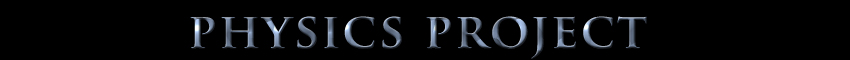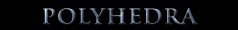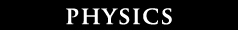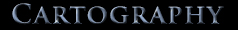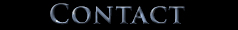## Waterman Physics

 Mathematical logic of the Galilean equation x' = x-vt challenged...where x = distance and (x,y,z) = a point Given Cartesian coordinate system S(x,y,z), the abscissa x mathematically means the lineal distance along the x axis from S(0,0,0) to S(x,0,0). Given Cartesian coordinate system S'(x',y',z'), the abscissa x' mathematically means the lineal distance along the x' axis from S'(0,0,0) to S'(x',0,0). Given that the abscissa x of S equals the abscissa x' of S'; [x' = x] when both systems are coincident, then it is true, that should either system be moved from that coincidence a distance equal to vt, that the abscissa of S = abscissa of S'; [x' = x], therefore [the Galilean transformation equation abscissa of x' = abscissa of x -vt is incorrect]. Noting too that S'(x',y',z') = S(x-vt,y,z) is true.

WHAT IF coordinates ARE fixed?
math versus physical
fixed point space
fixed interval time

WHAT IF matter IS solely composed of "unit/fixed" spheres of equal size and equal mass?
fixed quanta mass

WHAT IF photons ARE clusters of unit spheres?
fixed wavelength light

17 postulates [ time, space, light, mass ]

establishing a reference system

my challenge to the mathematics of Relativity    [ 2 xkcd threads combined totals of over 6000 posts and over 280,000 views from July 2012 ]

This applet among several applets written during the thread by Robert Schroll employs my intersection algorithms to determine
THE transmission site-and-time when applying a universal time approach.
I am postulating that this approach could manifest BOTH the simultaneous location AND the momentum of a particle.

FTL Effect

11 million mile tall dancer

original poems sparked by xkcd comics      chess photo     a bunch of rocks     purity

Payment may be done by Interac e-mail transfer or by using PayPal.
[ you do not need to be a PayPal member to do this....just my email address (swaterman@watermanpolyhedron.com)]

HOME